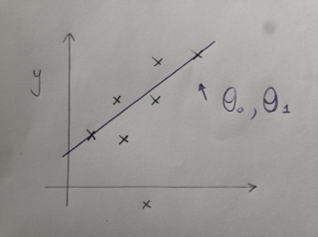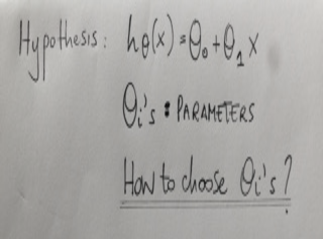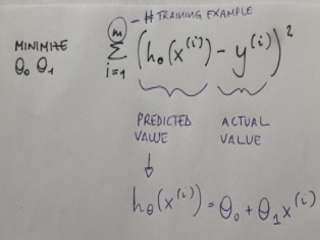## Machine Learning 101: the Cost Function or Squared Error Function

by Benedetta Tagliaferri

Welcome back to Machine Learning 101!

Today I am going to speak about the cost function, in other words how do we choose the right parameters that best fit our model.

Just to remark some fundamental concepts, in linear regression we have a training set and what we want to come up with values for our parameters so that the straight line that we create between our points is the one that best fits our data.But how we come up with parameters that best fit our data?Ideally we want to choose parameters so that h(x) is really close to y for our training example (x,y).

In our training sample we give a number of examples (remember the house prices by size in the previous blog, we know the size and the correspondent price), so let’s try to choose values of the parameters where at least on the training set given the x we match the y predicted values.

In other words I want to minimize the difference between the prediction and the actual price of the houses.So let’s explain this:

we are saying find me the values of this parameters that minimize the expression on blue, so that the sum of squared errors between the predicted value and the actual value is as small as possible (minimized).

So my cost function is also called squared error function.

Why we take the squared of the errors?

It is the most commonly used for regression problems.

In my previous blog about predicted Sales, I used the cost function in order to decide between linear regression model, boosted model and Spline model.

I hope this is useful!Benedetta Tagliaferri

Thu 03 Mar 2016

Thu 25 Feb 2016

Thu 18 Feb 2016

Thu 18 Feb 2016

© 2021 The Information Lab Ltd. All rights reserved.